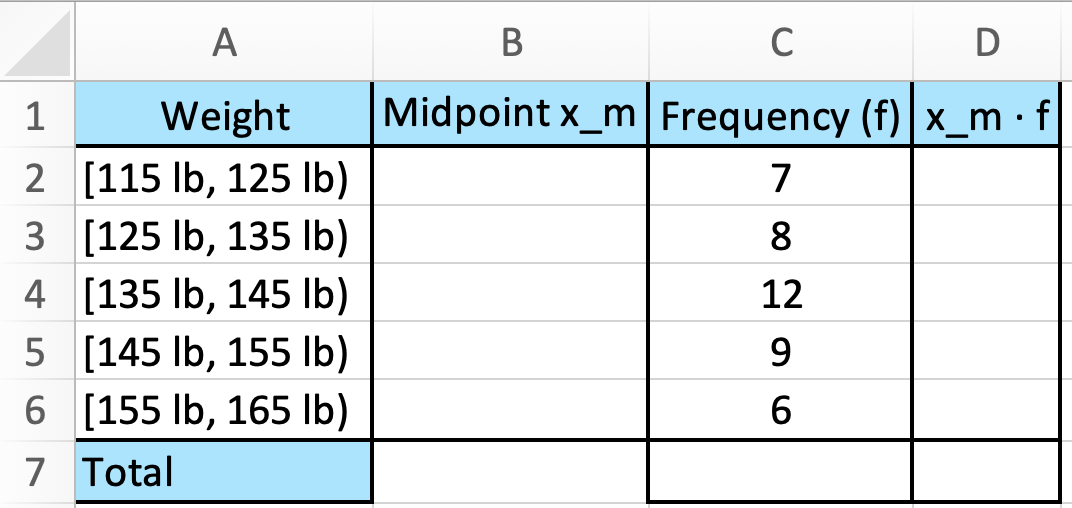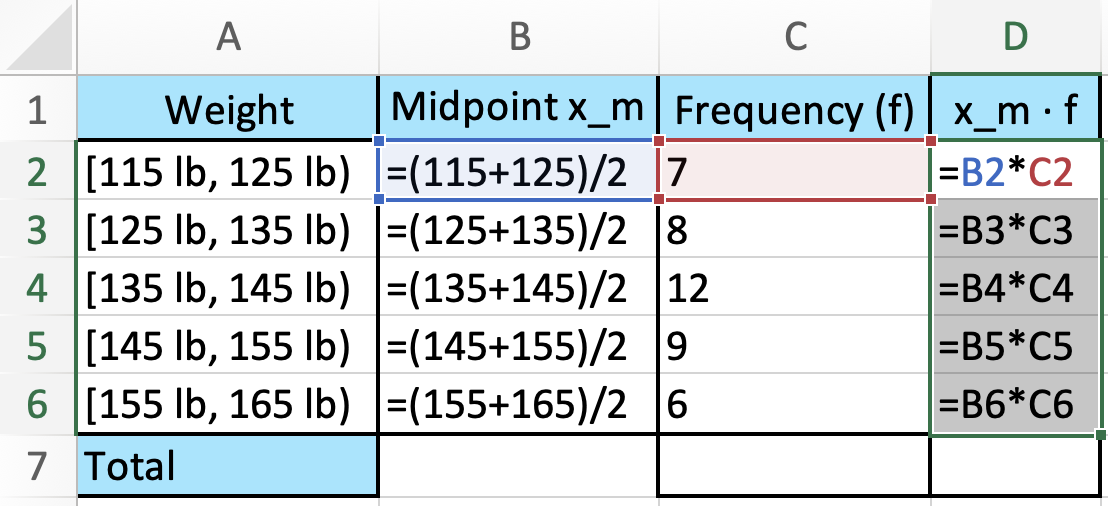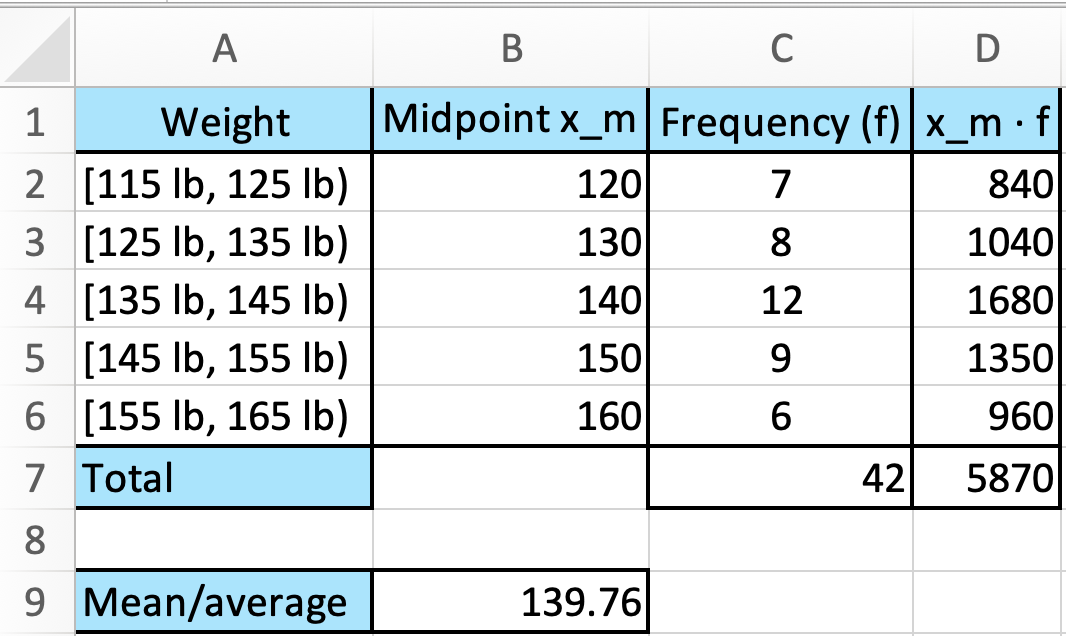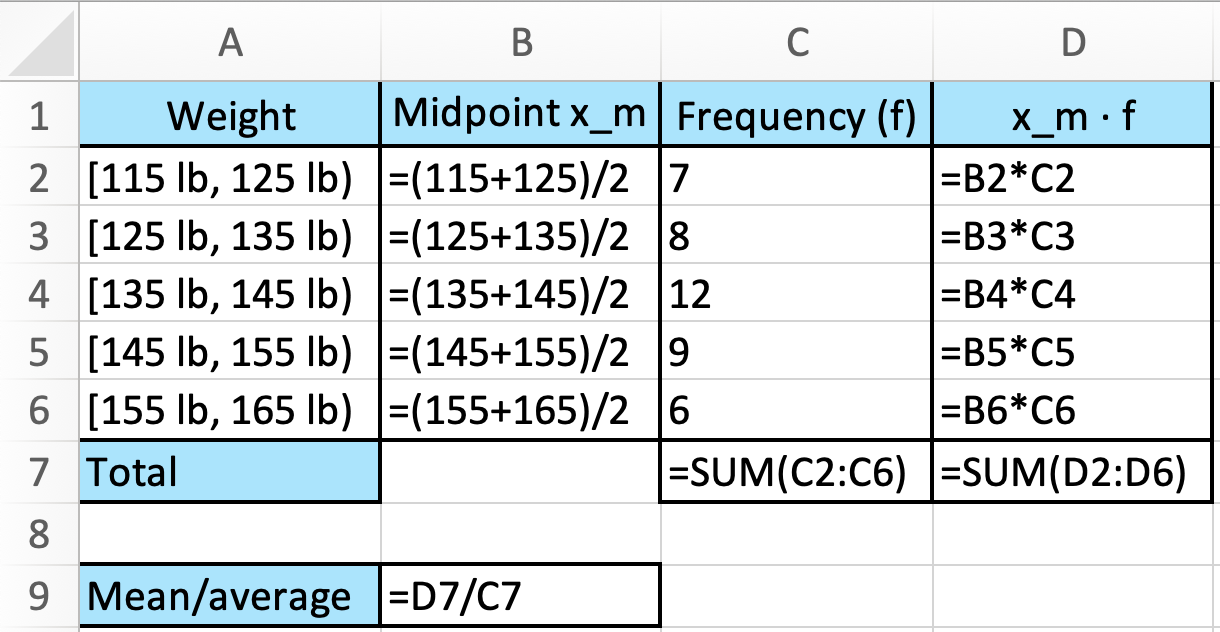# How to Find Mean of Grouped Data in Excel

`Excel` Instruction

### FindingtheMean of Grouped Data

1.
Make a frequency table in `Excel`.
2.
Add two new columns: One for the midpoint of each group, and one for the product of the midpoint and the frequency.
3.
Enter the formulas in the two new columns.
4.
Find the sum of the column representing the product of the midpoint and the frequency, and divide this by the sum of the frequency.

Now you have found the mean!

Example 1

A group of skaters are distributed into different weight classes. Here’s a table showing the classes and the frequency of each class.

 Weight Frequency $\phantom{\rule{-0.17em}{0ex}}\left[\text{}115\text{}\phantom{\rule{0.17em}{0ex}}\text{lb}-\text{}125\text{}\phantom{\rule{0.17em}{0ex}}\text{lb}\right)$ 7 $\phantom{\rule{-0.17em}{0ex}}\left[\text{}125\text{}\phantom{\rule{0.17em}{0ex}}\text{lb}-\text{}135\text{}\phantom{\rule{0.17em}{0ex}}\text{lb}\right)$ 8 $\phantom{\rule{-0.17em}{0ex}}\left[\text{}135\text{}\phantom{\rule{0.17em}{0ex}}\text{lb}-\text{}145\text{}\phantom{\rule{0.17em}{0ex}}\text{lb}\right)$ 12 $\phantom{\rule{-0.17em}{0ex}}\left[\text{}145\text{}\phantom{\rule{0.17em}{0ex}}\text{lb}-\text{}155\text{}\phantom{\rule{0.17em}{0ex}}\text{lb}\right)$ 9 $\phantom{\rule{-0.17em}{0ex}}\left[\text{}155\text{}\phantom{\rule{0.17em}{0ex}}\text{lb}-\text{}165\text{}\phantom{\rule{0.17em}{0ex}}\text{lb}\right)$ 6

Find the mean weight of this group.

1.
You can see the frequency table of the weight distribution above. The first thing to do is to enter the numbers in `Excel`.
2.
Add the columns “Midpoint ${x}_{m}$” and “${x}_{m}\cdot f$”. The last column is the product of the midpoint and the frequency. Your table should now look like this:3.
As you don’t know the exact weight of everyone in the weight class (it could be anywhere between $115$ lb-$125$ lb, $125$ lb-$135$ lb, etc.) you have to assume that the weight of everyone in each weight class is in the exact middle. This would be the average of the numbers 115 and 125 for the first, 125 and 135 for the second, and so on.

Write the following formula in cell `B2`:

=(115+125)/2

Enter the formula for calculating the mean in the remaining weight classes in the column representing the midpoints.

In the “${x}_{m}\cdot f$”-column, write the following formula in cell `D2`:

=B2*C2

Mark cell `D2` and copy the formula all the way down to cell `D6`.4.
Now find the sum of the numbers in the frequency and “${x}_{m}\cdot f$” columns. Write the following in cell `C7`:

=SUM(C2:C6)

And write the following in cell `D7`:

=SUM(D2:D6)

Finally, you’ll find the mean by dividing the number in cell `D7` by cell `C7`.

In cell `B9`, write:

=D7/C7Here are the formulas for each cell:You can now see that the mean weight for this group is $139.76$ lb.